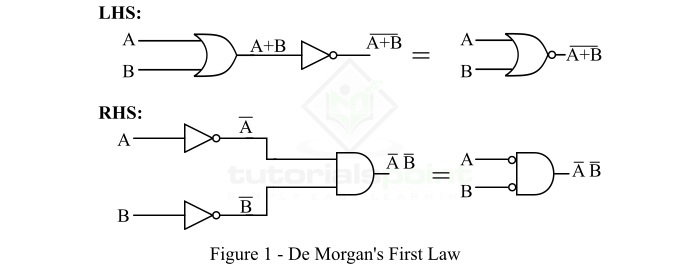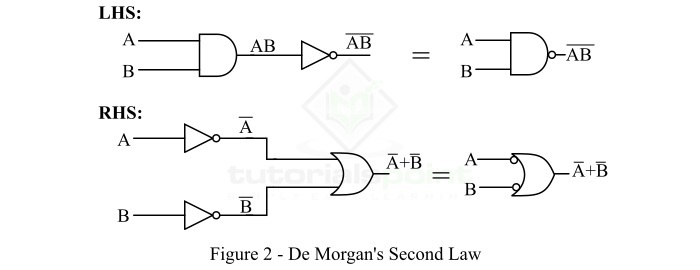# De Morgan's Theorem in Dual Form

In Boolean algebra, several rules are defined to perform operations in digital logic circuits. Boolean algebra is a tool to perform operation on binary digits, i.e. 0 and 1. These two binary digits 0 and 1 are used to denote FALSE and TRUE states of a digital circuit at input and output ends. Boolean algebra, developed by George Boole, uses 0s and 1s to create truth tables and logic expressions of digital circuits like AND, OR, NOT, etc. which are used to analyze and simplify the complex circuits.

There were another English mathematician Augustus De Morgan who explained the NAND and NOR operations as NOT AND and NOT OR operations respectively. This explanation was named De Morgan's Theorem. In this tutorial, we will discuss the De Morgan's theorem in detail.

## What is De Morgan's Theorem?

De Morgan's Theorem is a powerful theorem in Boolean algebra which has a set of two rules or laws. These two laws were developed to show the relationship between two variable AND, OR, and NOT operations. These two rules enable the variables to be negated, i.e. opposite of their original form. Therefore, De Morgan's theorem gives the dual of a logic function.

Now, let us discuss the two laws of De Morgan's theorem.

## De Morgan's First Theorem (Law 1)

De Morgan's First Law states that the complement of a sum (ORing) of variables is equal to the product (ANDing) of their individual complements. In other words, the complement of two or more ORed variables is equivalent to the AND of the complements of each of the individual variables, i.e.

$$\mathrm{\overline{A+B}=\overline{A}. \overline{B} }$$

Or, it may also be represented as,

$$\mathrm{\lgroup A+B\rgroup'= A' .B'}$$

The logic implementation of left side and right side of this law is shown in Figure 1.Thus, De Morgan's first law proves that the NOR gate is equivalent to a bubbled AND gate. The following truth table shows the proof of this law.

Left Side

Right Side

Inputs

Output

Inputs

Output

A

B

(A+B)'

A'

B'

A'.B'

0

0

1

1

1

1

0

1

0

1

0

0

1

0

0

0

1

0

1

1

0

0

0

0

This truth table proves that the Boolean expression on the left is equivalent to that on the right side of the expression of De Morgan's first law.

Also, the first law of De Morgan's theorem can be extended to any number of variables, or a combination of variables.

For example,

$$\mathrm{\overline{A+B+C+D+E+\dotso}=\overline{A}\: \overline{B}\: \overline{C}\: \overline{D}\:\overline{E}\:\dotso}$$

Also,

$$\mathrm{\overline{ABC+DE+FGH+\dotso}=\overline{\lgroup ABC\rgroup}.\overline{\lgroup DE\rgroup}.\overline{\lgroup FGH\rgroup}.\dotso}$$

From the above discussion, we may conclude that the De Morgan's First Law converts an expression from a sum form under a NOT sign to a product form.

## De Morgan's Second Theorem (Law 2)

De Morgan's second law states that the complement of the product (ANDing) of variables is equivalent to the sum (ORing) of their individual complements. In other words, the complement of two or more ANDed variables is equal to the sum of the complement of each of the individual variables, i.e.,

$$\mathrm{\overline{AB}=\overline{A}+\overline{B}}$$

It may also be represented as,

$$\mathrm{\lgroup AB\rgroup'= A' +B'}$$

The logic implementation of left and right sides of this expression is shown in Figure 2.Hence, De Morgan's second law proves that the NAND gate is equivalent to a bubbled OR gate. The following truth table shows the proof of this law.

Left Side

Right Side

Inputs

Output

Inputs

Output

A

B

AB

A'

B'

A'+B'

0

0

0

1

1

1

0

1

1

1

0

1

1

0

1

0

1

1

1

1

1

0

0

0

This truth table proves that the Boolean expression on the left side is equivalent to that on the right side of the expression of De Morgan's second law.

Similar to the first law, we may extend the De Morgan's second law for any number of variables or combination of variables.

For example,

$$\mathrm{\overline{ABCDE\dotso}= \overline{A} +\overline{B}+\overline{C}+\overline{D}+\overline{E}+\dotso}$$

And, for a combination of variables,

$$\mathrm{\overline{\lgroup ABC\rgroup}\overline{\lgroup DE\rgroup}\overline{\lgroup FG\rgroup\dotso}= \overline{ABC} +\overline{DE}+\overline{FG}}$$

Hence, from the above discussion, we can conclude that De Morgan's second law transforms a product form of variables or combination of variables under a NOT sign into a sum form.

Therefore, De Morgan's laws transforms an AND operation into an OR operation, and an OR operation into an AND operation. This principle is called duality.

## Example 1

Apply De Morgan's theorem to the following Boolean expression,

$$\mathrm{F=\overline{AB\overline{\lgroup C+D\rgroup}EF}}$$

### Solution

Given expression is,

$$\mathrm{F=\overline{AB\overline{\lgroup C+D\rgroup}EF}}$$

As the given expression has AND operation under a NOT sign, thus on applying De Morgan's second law, we get,

$$\mathrm{F=\overline{AB}+\lgroup C+D\rgroup+\overline{EF}}$$

This is the equivalent or the dual of the given expression.

## Example 2

Apply De Morgan's theorem to the following Boolean expression,

$$\mathrm{F=\overline{AB+\overline{CD}}}$$

### Solution

Given expression is,

$$\mathrm{F=\overline{AB+\overline{CD}}}$$

The given expression is in the form of a sum of variables under a NOT sign, thus on applying De Morgan's first law, we get the dual of this expression.

$$\mathrm{F=\overline{AB}\cdot\overline{\overline{CD}}=\overline{AB}\cdot CD}$$

## Conclusion

In this tutorial, we explained the two laws of De Morgan's Theorem and showed how they are helpful in performing different operations in digital logic circuits.

Updated on: 03-Oct-2023

53 Views